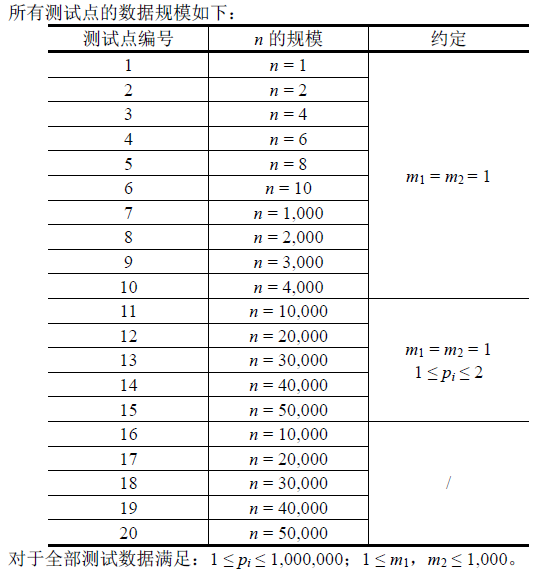# 2017.2.12【初中部 GDKOI】模拟赛B组 T2:宿敌

**【NOIP2014模拟7.10】宿敌(enemy) **
2014 年到了，T国和S 国在紧张交战中，现在到了最后一战。T国是一个实力很强的国家，在T国中，有一批为作战献身的机器人，对于任意一个机器人，有一个属性值pi，这个值越大，表示实力越强。T国将准备一支由n 个机器人组成的队伍，按照编号依次排开。

Input

Output

Sample Input

5 1 1

1 3 2 2 1

Sample Output

1

【样例说明】

Data ConstraintO(n^2)

type
new = array [0..50000] of longint;
var
i,j,k,l,n,m,m1,m2,t,ans:longint;
f,g:array[0..50000,1..1000] of longint;
a,d:new;
procedure discretize (var a : new); //离散化主程序
var
i,j,k:longint;
b, c : new;
procedure qsort (l, r : longint);
var
x, y : longint;
i, j : longint;
begin
i := l;
j := r;
x := a [(l + r) div 2];
repeat
while a [i] < x do inc (i);
while x < a [j] do dec (j);
if not (i > j) then
begin
y := a [i];
a [i] := a [j];
a [j] := y;
y := b [i];
b [i] := b [j];
b [j] := y;
inc (i);
dec (j);
end;
until i > j;
if l < j then qsort (l, j);
if i < r then qsort (i, r);
end;
begin
fillchar (b, sizeof (b), 0);
fillchar (c, sizeof (c), 0);
for i := 0 to n do b [i] := i;
qsort (0, n);
j:=0;
c [b ] := 0;
for i := 1 to n do
if (a [i] = a [i - 1]) then
begin
c [b [i]] := c [b [i - 1]];
end
else
begin
inc(j);
c [b [i]] := j;
end;
fillchar (a, sizeof (a), 0);
for i := 0 to n do
begin
if c[i]>t then t:=c[i];
a [i] := c [i];
end;
end;
function min(x,y:longint):longint;
begin
if x>y then exit(y) else exit(x);
end;
function max(x,y:longint):longint;
begin
if x<y then exit(y) else exit(x);
end;
function chang(x,y:longint):longint;
begin
if x<y then exit(m1)
else if x>y then exit(m2)
else exit(0);
end;
begin
assign(input,'enemy.in');reset(input);
assign(output,'enemy.out');rewrite(output);
for i:=1 to n do
begin
end;
discretize(a);
for i:=1 to n do
begin
k:=maxlongint;
for j:=1 to t do
begin
k:=min(k,f[i-1,j]);
f[i,j]:=k+chang(a[i],j);
end;
end;
ans:=maxlongint;
for i:=1 to t do
ans:=min(f[n,i],ans);
j:=n;
d:=a;
for i:=1 to n div 2 do
begin
d[i]:=a[j];
d[j]:=a[i];
dec(j);
end;
for i:=1 to n do
begin
k:=maxlongint;
for j:=1 to t do
begin
k:=min(k,g[i-1,j]);
g[i,j]:=k+chang(d[i],j);
end;
end;
for i:=1 to t do
ans:=min(g[n,i],ans);
writeln(ans);
close(input);close(output);
end.